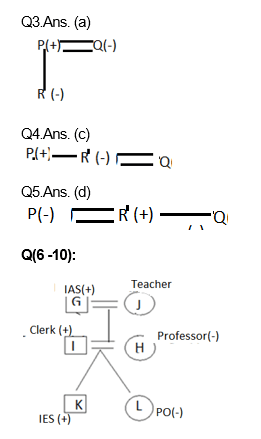# Reasoning Quiz for IBPS CLERK MAINS 2018| 13th January 2019

Reasoning Questions and Reasoning Quiz for IBPS PO Reasoning , IBPS Clerk Reasoning , IBPS RRB Reasoning ,SBI exam Reasoning , and other competitive exams.

Q1. Sunny walked 6 meters facing towards East, then took a right turn and walked a distance of 9 meters. He then took a left turn and walked a distance of 6 meters. How far is he from the starting point ?

(a) 15 meters

(b) 21 meters

(c) 18 meters

(d) Can’t be determined

(e) None of these

Q2. Ashish walks 10 meters towards the South. Turning to the left, he walks 20 meters and then moves to his right. After moving a distance of 20 meters, he turns to the right and walks 20 meters. Finally, he turns to the right and moves a distance of 10 meters. How far and in which direction is he from the starting point ?

(a) 10 meters North

(b) 20 meters South

(c) 20 meters North

(d) 10 meters South

(e) None of these

Directions (Q3-Q5): Read the following information carefully and then answer the questions given below it:

A + B means A is the father of B, A – B means A is the wife of B; A × B means A is the brother of B; A ÷ B means A is the daughter of B.

Q3. If P + R ÷ Q, which of the following is true ?

(a) P is the husband of Q

(b) P is the brother of Q

(c) P is the son of Q

(d) P is the father of Q

(e) None of these

Q4. If P × R – Q,  which of the following is true ?

(a) P is the Sister of Q

(b)P is the brother of Q

(c)P is the brother-in-law of Q

(d) P is the uncle of Q

(e) None of these

Q5. If P – R × Q, which of the following is true ?

(a) P is the brother of Q

(b) Q is the son of P

(c) Q is the husband of P

(d) P is the sister-in-law of Q

(e) None of these

Directions (Q6-Q10): Read the following information carefully and answer the questions given below it.

There is a family living in apartment in Ghaziabad and their six persons i.e. G, H, I, J, K and L, have different profession i.e. PO, Teacher, Professor, Clerk, IAS and IES.

The one who is IAS is the grandfather of L, who is a PO. The Teacher J is married to G. I, the Clerk, is married to the Professor. H is the mother of L and K. There are two married couples in the family. The PO is a female while IES is a male.

Q6. What is the profession of K?

(a) IAS

(b) IES

(c) Teacher

(d) PO

(e) None of these

Q7. How is G related to K?

(a) Brother

(b) Uncle

(c) Father

(d) Grandfather

(e) None of these

Q8. How many male members are there in the family?

(a) One

(b) Three

(c) Four

(d) Two

(e) None of these

Q9. What is the profession of G?

(a) IAS

(b) Professor

(c) Clerk

(d) Teacher

(e) None of these

Q10. Which of the following is one of the pair of couples in the family?

(a) GH

(b) GI

(c) GJ

(d) GK

(e) None of these

# SolutionsQ6. Ans.(b)

Q7. Ans.(d)

Q8. Ans.(b)

Q9. Ans.(a)

Q10. Ans.(c)

## WhatsApp Group Join here

Mail us at : ambitiousbaba1@gmail.com